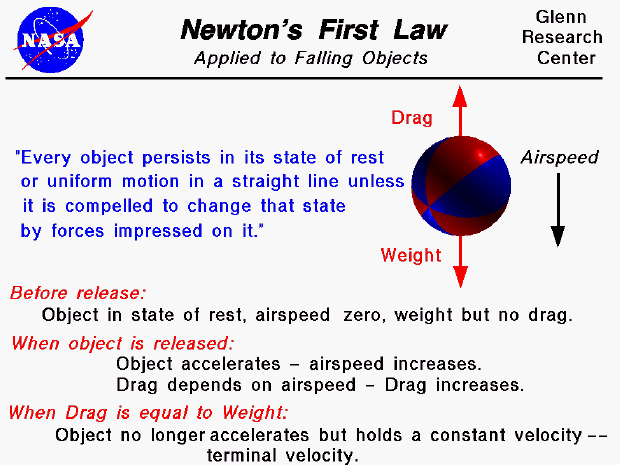Sir Isaac Newton first presented his three laws of motion in the "Principia Mathematica Philosophiae Naturalis" in 1686. His first law states that every object will remain at rest or in uniform motion in a straight line unless compelled to change its state by the action of an external force. This is normally taken as the definition of inertia. The key point here is that if there is no net force acting on an object (if all the external forces cancel each other out) then the object will maintain a constant velocity. If that velocity is zero, then the object remains at rest. And if an additional external force is applied, the velocity will change because of the force.

An object falling through the atmosphere is a good example of this principle. Just prior to release, the velocity of the object is zero, the object is at rest, and the weight of the object is balanced by some restraining device (a rope). There is no net force on the object, and the object would remain at rest indefinitely. When the rope is cut, the object is subjected to a single force, the gravitational attraction of the earth. Since there is no initial air resistance, the object begins to free fall and accelerate. But as the object velocity increases, it encounters air resistance, or drag, which opposes the motion. The magnitude of the drag depends on the square of the velocity. The drag increases until it is equal to the weight. At that point, there is no net external force on the object, the acceleration goes to zero, and the body falls at a constant terminal velocity. The magnitude of the velocity depends on the relative magnitude of the weight, the drag coefficient, the air density, and the size of the object.

Activities:

Guided Tours